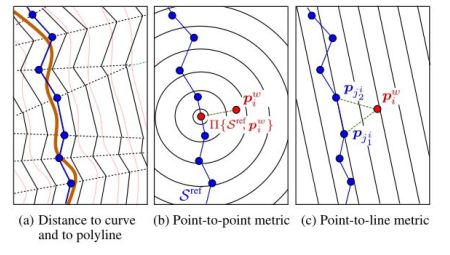0%

## PL-ICP 匹配方法

### 基本思想### 数学描述

$S^{ref}$ 代表参考激光帧生成曲面上的投影，这里我的理解是由于原始 ICP 没有考虑曲面的影响而是直接匹配每一个点，所以对该点处曲面的投影就是该点，所以对 ICP 而言，$||\mathbf{p}_i \oplus \mathbf{q} - \prod{S^{ref}, \mathbf{p}_i \oplus \mathbf{q}}|| = ||\mathbf{p}_i \oplus \mathbf{q} - x_i||, x_i$ 就是匹配点。

PL-ICP 目标函数如下：

### 求解过程 & 具体算法

• 参考激光帧 $y_{t-1}$
• 当前激光帧 $y_t$
• 参考激光帧生成的曲面 $S^{ref}$
• 初始解 $q_o$

• 将当前帧的数据根据初始位姿 $q_k$ 投影到参考帧坐标系下
• 对于当前帧的点 $i$， 在参考帧中找到最近的两个点（因为要连成直线）$(j_1, j_2)$
• 计算直线误差，去除误差过大的点
• 最小化误差函数 $\sum{i}(\mathbf{n}^T[\mathbf{R}(\theta)\mathbf{p} + \mathbf{t} - p{j_1^i}])^2$

### 跟 ICP 的区别

PL-ICP 的求解精度更高（更符合实际），尤其是在人工结构化环境中（直线多）；

PL-ICP 对初值更敏感，通常要结合里程计一起使用

## NICP 匹配方法

### NICP 数学描述

$p_i$ 表示激光点坐标 $(x, y, z)$

$n_i$ 表示对应点的法向量

$\sigma_i$ 对应点曲率

$\tilde{p}_i = (p_i, n_i)^T$ 为扩展点（坐标加法向量）

$T$ 表示欧式变换矩阵

$\oplus$ 对于 $\tilde{p}$ 的操作符（对于法向量只需要考虑旋转）：

## IMLS-ICP 匹配方法 （Implicit Moving Least Square）

### 曲面重建

$I^{P_k}(x) = 0$ 对应的集合表示曲面，空间中点到曲面的距离代入方程计算即可，证明过程看参考文献 Provably good moving least square

### 匹配求解

• 当前帧中一点 $x_i$ 到曲面的距离为$I^{P_k}(x_i)$
• $P_k$ 中离点 $x_i$ 最近点的法向量为 $n_i$
• 点 $x_i$ 在曲面的投影为：$y_i = x_i - I^{P_k}(x_i)n_i$
• 点 $x_i$ 和 点 $y_i$ 为对应的匹配点，求解误差： $\sum((Rx_i + t - y_i)n_i)^2$